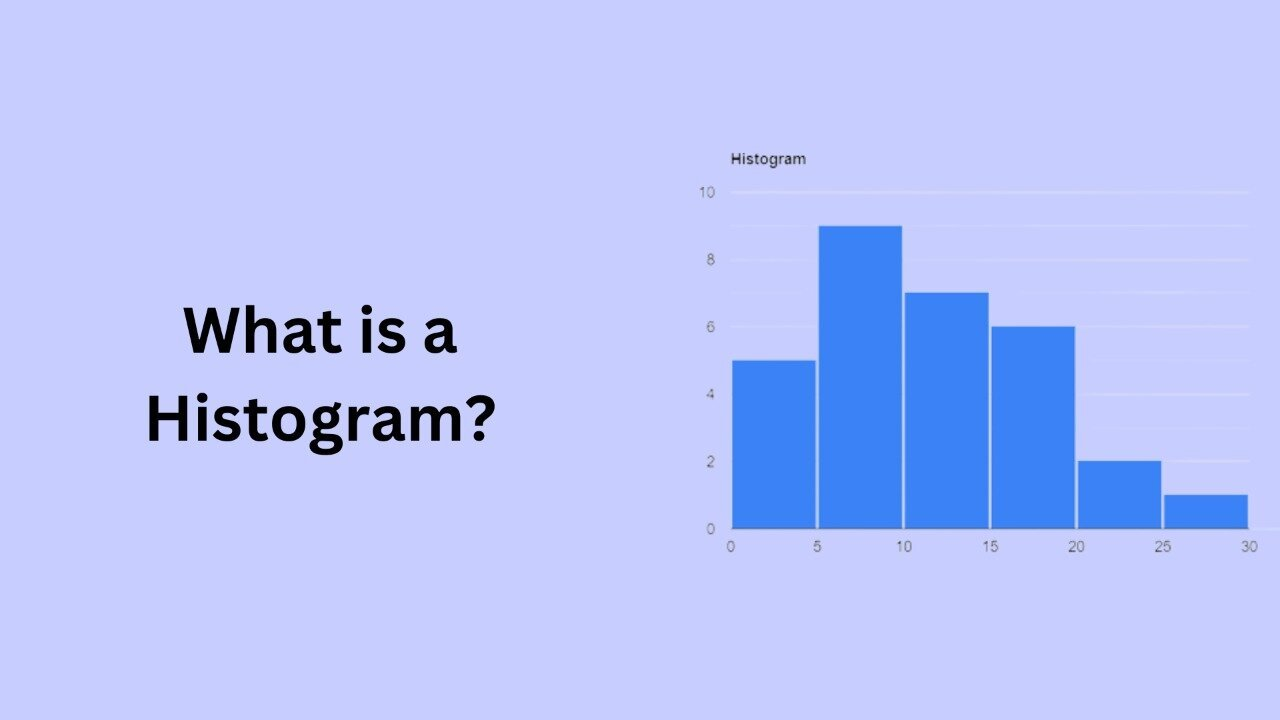# What is a Histogram?A histogram is a graphical representation of the distribution of numerical data. It is a type of bar chart that shows the frequency or number of observations within different numerical ranges called bins. Bins are usually specified as consecutive, non-overlapping intervals of a variable. A histogram provides a visual representation of the data distribution and shows the number of observations that fall into each bin.

Histograms are useful tools for visualizing distributions. Analyze numerical data and identify patterns and trends in the data. They are widely used and standard in statistical analysis. A feature of many statistical software packages.

To create a histogram, you first need to specify the bins or intervals that will be used to group the data. Sections should be chosen to accurately reflect the distribution of the data and allow the identification of patterns or trends in the data.

Another type of data that is well suited for histograms is data that is one-sided or has long tails. A skewed distribution is one that is not symmetrical and has longer tails on one side than the other. Histograms are useful tools for visualizing skewed distributions, as they show the frequency of observations in different bins and allow you to see the shape of the distribution and the presence of outliers.

Once the bins are specified, you can count the number of observations that fall within each bin, and create a bar for each bin on the histogram. The height of each bar represents the frequency or number of observations within that bin.

A histogram can be used to visualize the distribution of a single numerical variable, or to compare the distribution of multiple numerical variables. It can also be used to identify outliers in the data, or to identify clusters or gaps in the data.

In addition to visualizing the data, histograms can also be used to calculate summary statistics, such as the mean, median, and mode of the data. This can be useful for understanding the data and for making decisions based on the data.